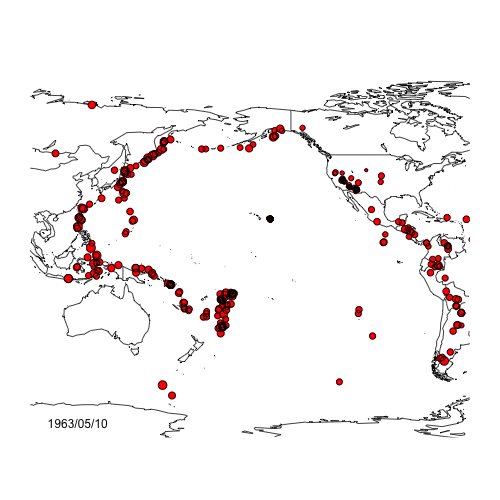# Talk on bivariate count times series in finance and risk management

I will be giving a talk on May 4th, at the Mathematical Finance Days, at HEC Montréal, on multivariate dynamic models for counts. The conference is organized by IFM2 (Institut de Finance Mathématique de Montréal). I will be chairing some session and I will give a talk based on the joint paper with Mathieu Boudreault.View more presentations from charthur.

In various situations in the insurance industry, in finance, in epidemiology, etc., one needs to represent the joint evolution of the number of occurrences of an event. In this paper, we present a multivariate integer‐valued autoregressive (MINAR) model, derive its properties and apply the model to earthquake occurrences across various pairs of tectonic plates. The model is an extension of Pedelis & Karlis (2011) where cross autocorrelation (spatial contagion in a seismic context) is considered. We fit various bivariate count models and find that for many contiguous tectonic plates, spatial contagion is significant in both directions. Furthermore, ignoring cross autocorrelation can underestimate the potential for high numbers of occurrences over the short‐term. An application to risk management and cat‐bond pricing will be discussed.# Even odds

This evening, I found a nice probabilistic puzzle on http://www.futilitycloset.com/“A bag contains 16 billiard balls, some white and some black. You draw two balls at the same time. It is equally likely that the two will be the same color as different colors. What is the proportion of colors within the bag?”
To be honest, I did not understood the answer on the blog, but if we write it down, we want to solveLet us count: ifis the total number of balls, and ifis the number of whiteballs thenI.e. we want to solve a polynomial equation (of order 2) in, or to be more precise, inIfis equal to 16, thenis either 6 or 10. It can be visualized below

```> balls=function(n=16){
+ NB=rep(NA,n)
+ for(k in 2:(n-2)){
+ NB[k]=(k*(k-1)+(n-k)*(n-k-1))
+ }
+ k=which(NB==n*(n-1)/2)
+ if(length(k)>0){
+ plot(1:n,NB,type="b")
+ abline(h=n*(n-1)/2,col="red")
+ points((1:n)[k],NB[k],pch=19,col="red")}
+ return((1:n)[k])}
> balls()
  6 10```But more generally, we can seek other‘s and other pairs of solutions of such a problem. I am not good in arithmetic, so let us run some codes. And what we get is quite nice: ifadmits a pair of solutions, thenis the squared of another integer, say. Further, the difference betweenandis precisely. Andwill be one of the answers when the total number of balls will be. Thus, recursively, it is extremely simple to get all possible answers. Below, we haveand the difference betweenand,

```> for(s in 4:1000){
+ b=balls(s)
+ if(length(b)>0) print(c(s,b,diff(b)))
+ }
 9 3 6 3
 16  6 10  4
 25 10 15  5
 36 15 21  6
 49 21 28  7
 64 28 36  8
 81 36 45  9
 100  45  55  10
 121  55  66  11
 144  66  78  12
 169  78  91  13
 196  91 105  14
 225 105 120  15
 256 120 136  16
 289 136 153  17
 324 153 171  18
 361 171 190  19
 400 190 210  20
 441 210 231  21
 484 231 253  22
 529 253 276  23
 576 276 300  24
 625 300 325  25
 676 325 351  26
 729 351 378  27
 784 378 406  28
 841 406 435  29
 900 435 465  30
 961 465 496  31```

Thus, given, consider an urn withballs. We draw two balls at the same time. It is equally likely that the two will be the same color as different colors. Then the number of colors within the bag are respectivelyFinally, observe that the‘s are well known, from Pascal’s triangle,also known as triangular numbers,Maths can be magic, sometimes…